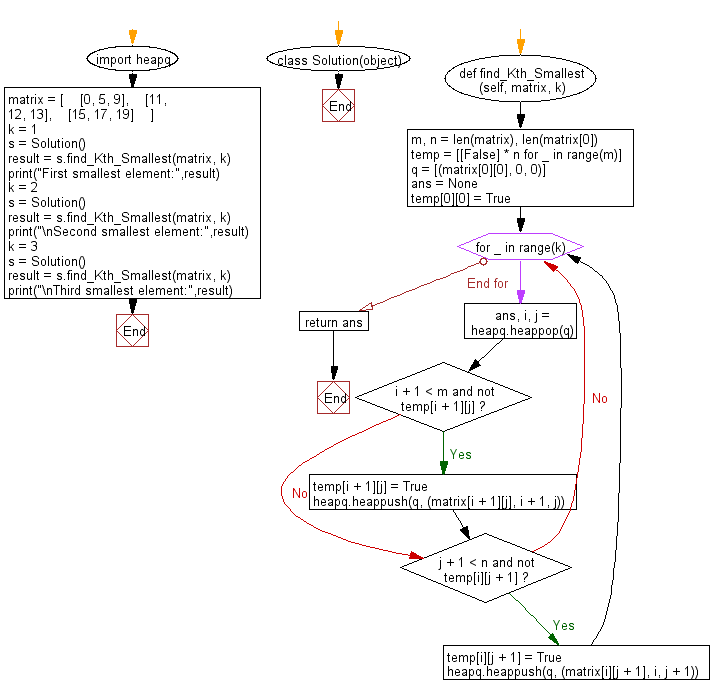﻿ Python heap queue algorithm: Find the kth smallest element in the matrix - w3resource

# Python: Find the kth smallest element in the matrix

## Python heap queue algorithm: Exercise-12 with Solution

Given a n x n matrix where each of the rows and columns is sorted in ascending order, write a Python program to find the kth smallest element in the matrix using the heap queue algorithm.

Assume k is always valid, 1 ≤ k ≤ n2 .

Sample Solution:

Python Code:

``````import heapq
class Solution(object):
def find_Kth_Smallest(self, matrix, k):
"""
:type matrix: List[List[int]]
:type k: int
:rtype: int
"""
m, n = len(matrix), len(matrix)
temp = [[False] * n for _ in range(m)]
q = [(matrix, 0, 0)]
ans = None
temp = True
for _ in range(k):
ans, i, j = heapq.heappop(q)
if i + 1 < m and not temp[i + 1][j]:
temp[i + 1][j] = True
heapq.heappush(q, (matrix[i + 1][j], i + 1, j))
if j + 1 < n and not temp[i][j + 1]:
temp[i][j + 1] = True
heapq.heappush(q, (matrix[i][j + 1], i, j + 1))
return ans

matrix = [
[0, 5, 9],
[11, 12, 13],
[15, 17, 19]
]
k = 1
s = Solution()
result = s.find_Kth_Smallest(matrix, k)
print("First smallest element:",result)
k = 2
s = Solution()
result = s.find_Kth_Smallest(matrix, k)
print("\nSecond smallest element:",result)
k = 3
s = Solution()
result = s.find_Kth_Smallest(matrix, k)
print("\nThird smallest element:",result)
```
```

Sample Output:

```First smallest element: 0

Second smallest element: 5

Third smallest element: 9
```

Flowchart:Python Code Editor:

Have another way to solve this solution? Contribute your code (and comments) through Disqus.

What is the difficulty level of this exercise?

Test your Programming skills with w3resource's quiz.

﻿